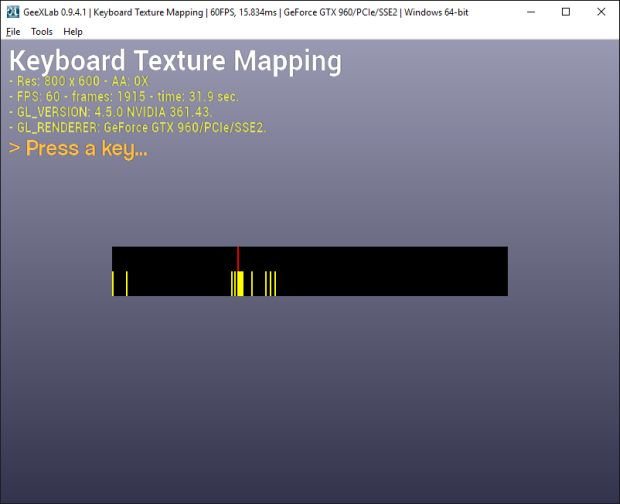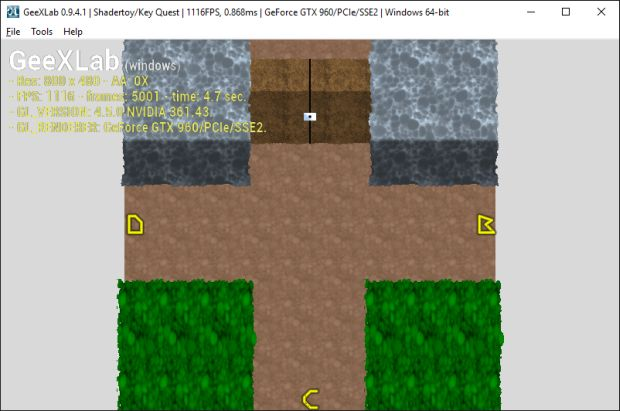Interesting question, especially if you try to port some Shadertoy demos that can read the keyboard from the pixel shader.

I’m going to describe a way to read the keyboard inside a shader that is directly compatible with Shadertoy demos.

The principle is actually quite easy: the keyboard buffer is mapped on a texture and this texture is read in the shader.

The keyboard buffer has 256 entries, one entry / key. The keyboard buffer is copied in a texture with 256×2=512 texels.

The first line of 256 texels is used to keep track of the toggled state: once a key, a texel of the second line will stay to 1 until the same key is pressed again. The second line of 256 texels is used to store the state of each key (pressed or released).

The first line can be sampled in the shader if uv.y < 0.5. The second line is sampled if uv.y >= 0.5.

Shadertoy uses Javascript key codes: the [backspace] key = 8, the [A] key = 65, and so on. Here is a web app that show the Javascript key codes: JavaScript Event KeyCodes.

GeeXLab virtual key codes are not the same than Javascript ones. So here is a code snipped in Lua that maps all GeeXLab key codes to Javascript ones:

```local lib_dir = gh_utils.get_scripting_libs_dir()
dofile(lib_dir .. "lua/keyboard_codes.lua")

g_keyboard_map = {}
for i=1, 256 do
g_keyboard_map[i] = 0
end

local js_backspace = 8
local js_tab = 9
local js_enter = 13
local js_shift = 16
local js_ctrl = 17
local js_alt = 18
local js_pause_break = 19
local js_caps_lock = 20
local js_escape = 27
local js_space = 8
local js_page_up = 33
local js_page_down = 34
local js_end = 35
local js_home = 36
local js_left_arrow =	37
local js_up_arrow =	38
local js_right_arrow = 39
local js_down_arrow = 40
local js_insert = 45
local js_delete = 46
local js_0 = 48
local js_1 = 49
local js_2 = 50
local js_3 = 51
local js_4 = 52
local js_5 = 53
local js_6 = 54
local js_7 = 55
local js_8 = 56
local js_9 = 57
local js_a = 65
local js_b = 66
local js_c = 67
local js_d = 68
local js_e = 69
local js_f = 70
local js_g = 71
local js_h = 72
local js_i = 73
local js_j = 74
local js_k = 75
local js_l = 76
local js_m = 77
local js_n = 78
local js_o = 79
local js_p = 80
local js_q = 81
local js_r = 82
local js_s = 83
local js_t = 84
local js_u = 85
local js_v = 86
local js_w = 87
local js_x = 88
local js_y = 89
local js_z = 90
local js_left_window_key = 91
local js_right_window_key = 92
local js_select_key = 93
local js_multiply = 106
local js_subtract = 109
local js_decimal_point = 110
local js_divide = 111
local js_f1 = 112
local js_f2 = 113
local js_f3 = 114
local js_f4 = 115
local js_f5 = 116
local js_f6 = 117
local js_f7 = 118
local js_f8 = 119
local js_f9 = 120
local js_f10 = 121
local js_f11 = 122
local js_f12 = 123
local js_num_lock = 144
local js_scroll_lock = 145
local js_semi_colon = 186
local js_equal_sign = 187
local js_comma = 188
local js_dash = 189
local js_period = 190
local js_forward_slash = 191
local js_grave_accent = 192
local js_open_bracket = 219
local js_back_slash = 220
local js_close_braket = 221
local js_single_quote = 222

g_keyboard_map[KC_A] = js_a
g_keyboard_map[KC_B] = js_b
g_keyboard_map[KC_C] = js_c
g_keyboard_map[KC_D] = js_d
g_keyboard_map[KC_E] = js_e
g_keyboard_map[KC_F] = js_f
g_keyboard_map[KC_G] = js_g
g_keyboard_map[KC_H] = js_h
g_keyboard_map[KC_I] = js_i
g_keyboard_map[KC_J] = js_j
g_keyboard_map[KC_K] = js_k
g_keyboard_map[KC_L] = js_l
g_keyboard_map[KC_M] = js_m
g_keyboard_map[KC_N] = js_n
g_keyboard_map[KC_O] = js_o
g_keyboard_map[KC_P] = js_p
g_keyboard_map[KC_Q] = js_q
g_keyboard_map[KC_R] = js_r
g_keyboard_map[KC_S] = js_s
g_keyboard_map[KC_T] = js_t
g_keyboard_map[KC_U] = js_u
g_keyboard_map[KC_V] = js_v
g_keyboard_map[KC_W] = js_w
g_keyboard_map[KC_X] = js_x
g_keyboard_map[KC_Y] = js_y
g_keyboard_map[KC_Z] = js_z

g_keyboard_map[KC_0] = js_0
g_keyboard_map[KC_1] = js_1
g_keyboard_map[KC_2] = js_2
g_keyboard_map[KC_3] = js_3
g_keyboard_map[KC_4] = js_4
g_keyboard_map[KC_5] = js_5
g_keyboard_map[KC_6] = js_6
g_keyboard_map[KC_7] = js_7
g_keyboard_map[KC_8] = js_8
g_keyboard_map[KC_9] = js_9

g_keyboard_map[KC_LEFT] = js_left_arrow
g_keyboard_map[KC_UP] = js_up_arrow
g_keyboard_map[KC_RIGHT] = js_right_arrow
g_keyboard_map[KC_DOWN] = js_down_arrow

g_keyboard_map[KC_F1] = js_f1
g_keyboard_map[KC_F2] = js_f2
g_keyboard_map[KC_F3] = js_f3
g_keyboard_map[KC_F4] = js_f4
g_keyboard_map[KC_F5] = js_f5
g_keyboard_map[KC_F6] = js_f6
g_keyboard_map[KC_F7] = js_f7
g_keyboard_map[KC_F8] = js_f8
g_keyboard_map[KC_F9] = js_f9
g_keyboard_map[KC_F10] = js_f10
g_keyboard_map[KC_F11] = js_f11
g_keyboard_map[KC_F12] = js_f12

g_keyboard_map[KC_SPACE] = js_space
g_keyboard_map[KC_LEFT_SHIFT] = js_shift
g_keyboard_map[KC_RIGHT_SHIFT] = js_shift
g_keyboard_map[KC_ESCAPE] = js_escape
g_keyboard_map[KC_BACK] = js_backspace
g_keyboard_map[KC_TAB] = js_tab
g_keyboard_map[KC_RETURN] = js_enter
g_keyboard_map[KC_PGDOWN] = js_page_down
g_keyboard_map[KC_PGUP] = js_page_up
g_keyboard_map[KC_HOME] = js_home
g_keyboard_map[KC_END] = js_end
g_keyboard_map[KC_INSERT] = js_insert
g_keyboard_map[KC_DELETE] = js_delete

g_keyboard_map[KC_SUBTRACT] = js_subtract
g_keyboard_map[KC_MULTIPLY] = js_multiply
g_keyboard_map[KC_DIVIDE] = js_divide

g_keyboard_map[KC_NUMLOCK] = js_num_lock

```

The keyboard mapping is the first part of the problem and it’s solved now. The second part is to update the keyboard texture.

First thing, the keyboard texture creation:

```g_toggle_state = {}
g_keyboard_prev_state = {}
local PF_U8_RGBA = 3
local PF_U8_R = 11
local x_size = 256
local y_size = 2
local k = 0
keyboard_tex = gh_texture.create_2d(x_size, y_size, PF_U8_RGBA)
for i=0, 255 do
g_toggle_state[k+1] = 0
g_keyboard_prev_state[k+1] = 0
k = k + 1
for j=0, 255 do
gh_texture.set_texel_2d(keyboard_tex, i, j, 0, 0, 0, 255)
end
end

local SAMPLER_FILTERING_NEAREST = 1
local SAMPLER_FILTERING_LINEAR = 2
gh_texture.bind(keyboard_tex, 0)
gh_texture.bind(0, 0)
```

The g_keyboard_prev_state array allows to keep track of the previous state (pressed or released) of each key. The g_toggle_state keeps track of the toggled state of each key. The g_toggle_state array allows to simulate pusk-like buttons (the button keeps its pressed state until next time it’s pressed).

Now here is the function that is called every frame to update the keyboard texture:

```function set_keyboard_texture_v2(gxl_kb_code, state)
local k = g_keyboard_map[gxl_kb_code]
if (k == nil) then
return
end

local v = 0
if (state == 1) then
-- Pressed state
v = 255
end

-- Store the pressed or released state (second line of the texture).
local row = 1
gh_texture.set_texel_2d(keyboard_tex, k, row, v,  0, 0, 255)

-- Store the toggled state (first line of the texture).
row = 0

local prev_state = g_keyboard_prev_state[k+1]

if (state == 1 and prev_state == 0) then
g_keyboard_prev_state[k+1] = 1
local toggled = g_toggle_state[k+1]
if (toggled == 0) then
g_toggle_state[k+1] = 1
gh_texture.set_texel_2d(keyboard_tex, k, row, 255, 255, 0, 255)
else
g_toggle_state[k+1] = 0
gh_texture.set_texel_2d(keyboard_tex, k, row, 0, 0, 0, 255)
end
end

if (state == 0) then
g_keyboard_prev_state[k+1] = 0
end

end
```

In a FRAME script, this function can be called like that:

```local platform_windows = 1
local platform_osx = 2
local platform_linux = 3

if (gh_utils.get_platform() == platform_windows) then
gh_window.keyboard_update_buffer(0)
end

for i=0, 255 do
local kbcode = i
local state = gh_input.keyboard_is_key_down(kbcode)
set_keyboard_texture(kbcode, state)
end
```

The keyboard texture is bound like any texture and can be sampled in the shader.

I prepared a simple code sample that displays the keyboard texture:The yellow lines show the toggle states while red lines are the pressed/released states. The demo (keyboard_to_texture_gl2.xml) is available in the host_api/keyboard/ folder of the code sample pack.

I also prepared another demo (that should help Stefan) this time based on this Shadertoy demo:This demo (key_quest_gl2.xml) is available in the host_api/GLSL_ShaderToy/ folder of the code sample pack.

References